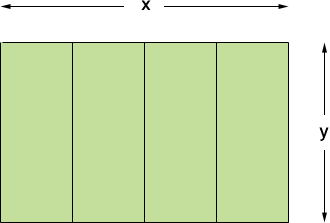SEARCH HOMEMath Central Quandaries & QueriesQuestion from Mark, a student: a rectangular field is to be enclosed and divided into four equal lots by fences parallel to one of the side. A total of 10000 meters of fence are available .Find the area of the largest field that can be enclosed.Hi Mark,

Draw a diagram!The measurements are in metres.

All together you need five lengths of length y meres each and two lengths of length x metres each. You have 10 000 metres of fencing so

5y + 2x = 10 000.

The area A is given by

A = xy square metres.

Solve the first equation for y and substitute into the second equation. Use the calculus you know to find the value of x that maximizes the area.

PennyMath Central is supported by the University of Regina and The Pacific Institute for the Mathematical Sciences.